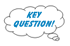# Lesson 3 : Linear RegressionPrinter-friendly version

### Introduction to Regression

 Key Learning Goals for this Lesson: Review of linear regression model focusing on prediction Use least square estimation for linear regression Apply model developed in training data to an independent test data Context setting for more complex supervised prediction methods Textbook reading: Consult Course Schedule

A quick review of regression, expectation, variance, and parameter estimation.

Input vector: $X = (X_1, X_2, ... , X_p)$.

Output Y is real-valued.

Predict Y from X by f(X) so that the expected loss function $E(L(Y, f(X)))$ is minimized.

### Review: Expectation

Intuitively, the expectation of a random variable is its "average" value under its distribution.

Formally, the expectation of a random variable X, denoted E[X], is its Lebesgue integral with respect to its distribution.

If X takes values in some countable numeric set $\chi$, then

$E(X) =\sum_{x \in \chi}xP(X=x)$

If $X \in \mathbb{R}^m$ has a density p, then

$E(X) =\int_{\mathbb{R}^m}xp(x)dx$

Expectation is linear: $E(aX +b)=aE(X) + b$.

Also, $E(X+Y) = E(X) +E(Y)$.

Expectation is monotone: if XY, then E(X) ≥ E(Y).

### Review: Variance

The variance of a random variable X is defined as:

$Var(X) = E[(X-E[X])^2]=E[X^2]-(E[X])^2$

and the variance obeys the following $a, b \in \mathbb{R}$:

$Var(aX + b) =a^2Var(X)$

### Review: Frequentist Basics

The data x1, ... , xn is generally assumed to be independent and identically distributed (i.i.d.).

We would like to estimate some unknown value θ associated with the distribution from which the data was generated.

In general, our estimate will be a function of the data (i.e., a statistic)

$\hat{\theta} =f(x_1, x_2, ... , x_n)$

Example:  Given the results of n independent flips of a coin, determine the probability p with which it lands on heads.

### Review: Parameter Estimation

In practice, we often seek to select a distribution (model) corresponding to our data.

If our model is parameterized by some set of values, then this problem is that of parameter estimation.

How can we obtain estimates in general?  One Answer:  Maximize the likelihood and the estimate is called the maximum likelihood estimate, MLE.

\begin {align} \hat{\theta} & = argmax_{\theta} \prod_{i=1}^{n}p_{\theta}(x_i) \\ & =argmax_{\theta} \sum_{i=1}^{n}log (p_{\theta}(x_i)) \\ \end {align}

Discussion

Let's look at the setup for linear regression. We have an input vector: X = (X1, X2, ..., Xp ). This vector is p dimensional.

The output Y is a real value and is ordered.

We want to predict Y from X.

Before we actually do the prediction we have to train the function f(X). By the end of the training, I would have a function f(X) to map every X into an estimated Y. Then, we need some way to measure how good this predictor function is. This is measured by the expectation of a loss.Why do we have loss in the estimation?

Y is actually a random variable given X. For instance, consider predicting someones weight based on the person's height. People can have different weights given the same height. If you think of the weight as Y and the height as X, Y is random given X. We therefore cannot have a perfect prediction for every subject because f(X) is a fixed function, impossible to be correct all the time. The loss measures how different the true Y is from your prediction.Why do we have the overall loss expressed as an expectation?

The loss may be different for different subjects. In statistics, a common thing to do is to average the losses over the entire population.

Squared loss:

L(Y, f(X)) = (Y - f(X))2 .

We simply measure the difference between the two variables and square them so that we can handle negative and positive difference symmetrically.

Suppose the distribution of Y given X is known, the optimal predictor is:

f*(X) = argminf(X) E(Y - f(X))2

= E(Y | X) .

This is the conditional expectation of Y given X. The function E(Y | X) is called the regression function.

#### Example

We want to predict the number of physicians in a metropolitan area.

Problem: The number of active physicians in a Standard Metropolitan Statistical Area (SMSA), denoted by Y, is expected to be related to total population (X1, measured in thousands), land area (X2, measured in square miles), and total personal income (X3, measured in millions of dollars). Data are collected for 141 SMSAs, as shown in the following table.

 i : 1 2 3 ... 139 140 141 X1 9387 7031 7017 ... 233 232 231 X2 1348 4069 3719 ... 1011 813 654 X3 72100 52737 54542 ... 1337 1589 1148 Y 25627 15389 13326 ... 264 371 140

Our Goal: To predict Y from X1, X2, and X3.

This is a typical regression problem.